# Two Sample Proportion Test with JMP

### What is the Two Sample Proportion Test?

The two sample proportion test is a hypothesis test to compare the proportions of one certain event occurring in two populations following the binomial distribution.

• Null Hypothesis(H0): p1 = p2
• Alternative Hypothesis(Ha): p1 ≠ p2

### Two Sample Proportion Test Assumptions

• The sample data drawn from the populations of interest are unbiased and representative
• There are only two possible outcomes in each trial for both populations: success/failure, yes/no, and defective/non-defective etc.
• The underlying distributions of both populations are binomial distribution
• When np ≥ 5 and np(1 – p) ≥ 5, the binomial distribution can be approximated by the normal distribution

### How the Two Sample Proportion Test Works

When np ≥ 5 and np(1 – p) ≥ 5, we use normal distribution to approximate the underlying binomial distributions of the populations.
Test Statistic

Where: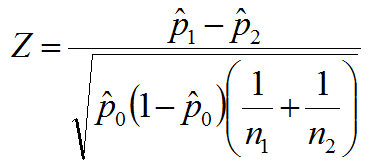and where: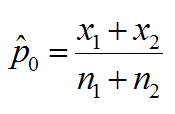•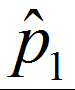and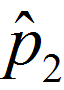are the observed proportions of events in the two samples
•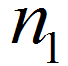and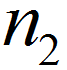is the number of trials in the two samples respectively
•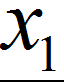and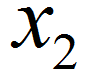is the number of events in the two samples respectively

When |Zcalc| is smaller than Zcrit, , we fail to reject the null hypothesis.

### Use JMP to Run a Two Sample Proportion Test

Case study: We are interested in comparing the exam pass rates of a high school in March and April using a nonparametric (i.e. distribution-free) hypothesis test: two sample proportion test.
Data File:“TwoSampleProportion.jmp”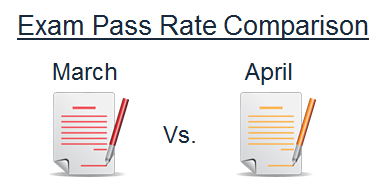• Null Hypothesis(H0): pMarch = pApril
• Alternative Hypothesis(Ha): pMarch ≠ pApril

#### Steps to run a two sample proportion test in JMP:

1. Click Analyze -> Fit Y by X
2. Select “Results” as “Y, Responses”
3. Select “Month” as “X, Factor”4. Click “OK”Model summary: The p-value of the two sample proportion test is 0.849, greater than the alpha level (0.05), and we fail to reject the null hypothesis. We conclude that the exam pass rates of the high school in March and April are not statistically different. In the output from the test we see the p-value is higher than the alpha value of 0.05; therefore, we fail to reject the null hypothesis and claim there is not a difference between the school’s exam pass rate in March and April.